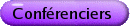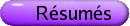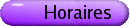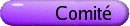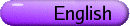Théorie des categories
Org: Robin Cockett (Calgary)
[PDF]

MICHAEL BARR, McGill University, Montreal, QC H3A 2K6
The category of Mackey spaces is *-autonomous
[PDF]

A standard theorem says that any locally convex topological vector space has a finer topology, its Mackey topology with the same set of continuous linear functionals and that is the finest possible topology with that property. If E and F are two such spaces, topologize the space Hom(E,F) of continuous linear transformations E® F with the weak topology induced by the algebraic tensor product EÄF¢ and then let [E,F] denote the associated Mackey topology. Let F* denote the dual F¢ topologized by the Mackey topology on the weak dual and let EÄF=[E,F*]* (whose underlying vector space is the algebraic tensor product). Then for any Mackey spaces E, F, and G,

1. [EÄF,G] @ [E,[F,G]]

2. E @ E* *

3. [E,F] @ (EÄF*)*

which is summarized by saying that the category of Mackey spaces and continuous linear transformations is *-autonomous.

This category is equivalent to the category of weakly topologized locally convex topological vector spaces (which have the coarsest possible topology for their set of continuous linear functionals) which is therefore also *-autonomous. They are also equivalent to the chu category of vector spaces (which will be explained).

MARTA BUNGE, McGill University, Dept. of Mathematics and Statistics, 805 Sherbrooke St. West, Montreal, QC H3A 2K6
Michael coverings are comprehensive
[PDF]

The motivational example for the comprehension scheme (Lawvere '68) came from proof theory. An example with categories as types (Gray '69, Street-Walters '73) exhibited comprehension as the familiar Grothendieck construction of a discrete opfibration associated with a covariant functor F : B ® Sets on a small category B.

We introduce the setting of an "extensive 2-doctrine" (E2D) in which to state the comprehension scheme in a 2-categorical setting. This involves a 2-category T "of types" and, for each object X of T, a category E(X) of "extensive quantities of type X" with a terminal object 1X, and a "pushforward operation" E(f) :E(Y) ® E(X) for each 1-cell f : Y ® X in T. For each object X of T, we have a 2-functor BX : (T,X) ® E(X) that assigns, to each 1-cell f : Y ® X, the extensive quantity of type X given by E(f)(1Y). We say that the E2D satisfies the comprehension scheme if for each X, the 2-functor BX has a fully faithful right 2-adjoint {-}B : E(X) ® (T,X), called comprehension. A 1-cell f : Y ® X is called E-dense if the canonical map E(f)(1Y) ® 1X is an isomorphism, and it is called an E-covering if the unit f ® {BX (f)}X is an isomorphism. It follows that every 1-cell f : Y ® X admits a unique (up to iso) factorization into an E-dense 1-cell Y ®Z, followed by an E-covering 1-cell Z ® X. This is called the "E-comprehensive factorization" of f.

The purpose of this talk is:

(1) to remark that the (pure, Fox complete spread) factorization (Bunge-Funk '96) is indeed comprehensive for an E2D with T the 2-category of locally connected (Grothendieck) toposes and E(X) the category of Lawvere distributions on X, and
(2) to prove that the (hyperpure, Michael Complete spread) factorization (Bunge-Funk 2005) is comprehensive for an E2D with T the 2-category of all (Grothendieck) toposes and E(X) a category of what we call "0-distributions", or distributions with values in 0-dimensional locales.

This is joint work with J. Funk.

JOHN FOUNTAIN, University of York, York YO10 5DD, UK
Proper covers of left ample monoids
[PDF]

The relation R* is defined on a monoid M by the rule that aR* b if and only if for all x,y Î M,

 xa = ya Û xb = yb.
If the set of idempotents E(M) of M is a commutative submonoid of M and every R*-class contains an idempotent, M is said to be left adequate. In such a monoid each R*-class contains a unique idempotent and the idempotent in the R*-class of an element a is denoted by af. A left adequate monoid M is left ample if ae = (ae)f a for all e Î E(M) and a Î M.

Thus a right cancellative monoid is left ample; here R* is the universal relation. Every inverse monoid is left ample.

A left ample monoid is proper if the intersection of the minimum left cancellative congruence and R* is trivial. The structure of proper left ample monoids can be described in terms of right cancellative monoids and commutative monoids of idempotents. Moreover, any left ample monoid M has a proper cover, that is, a proper left ample monoid P together with a homomorphism from P onto M which restricts to an isomorphism from E(P) onto E(M). We consider how such covers can be constructed.

PETER FREYD, Pennsylvania University
*-autonomous structures on old categories
[PDF]

Some anciently studied categories turn out to have *-autonomous structures not previously noted; for example, the category of finitely generated additive group-valued functors from finitely generated abelian groups. This is, in fact, the free abelian category on one object generator. As all free structures on one generator it has a monoidal structure (it may be identified as composition of functors). It is neither the tensor product nor the "par" but lies between them.

JONATHON FUNK, University of the West Indies, Cave Hill Campus
Semigroups and toposes
[PDF]

We shall present a strictly semigroup description of the classifying topos B(G)  of an inverse semigroup G. A left *-semigroup is a semigroup S together with an assignment s® s* satisfying:

(i) (s*)* = s,
(ii) ss*s = s, and
(iii) (s* st)* = (st)* s,
for all s,t Î S. A morphism of left *-semigroups is a function h : S® T such that

(i) h(s*) = h(s)*, and
(ii) h(st) = h(s)h(s* st).
Such a morphism h is said to be étale if every equation t=h(f)t in T, where f is a strong idempotent (f=f* f) of S, can be lifted uniquely to an equation s=fs in S, meaning h(s)=t.

Proposition 1 B(G) is equivalent to the category of étale morphisms of left *-semigroups over the inverse semigroup G.

We shall also present a strictly topos description of E-unitary inverse semigroups . A ØØ-separated object of a topos is one that is separated for the ØØ-topology in the topos . (F is ØØ-separated iff the diagonal subobject F\rightarrowtail F×F is equal to its double negation.)

Proposition 2 An inverse semigroup G is E-unitary iff the object d :G® E of B(G) is ØØ-separated, where E= idempotents of G, and d(t) = t* t.

## References


P. T. Johnstone, Sketches of an Elephant: A Topos Theory Compendium. Clarendon Press, Oxford, 2002.


A. Kock and I. Moerdijk, Presentations of étendues. Cahiers Topologie Géom. Différentielle Catég. (2) 32(1991), 145-164.


Mark V. Lawson, Inverse Semigroups: The Theory of Partial Symmetries. World Scientific Publishing Co., Singapore, 1998.

NICOLA GAMBINO, University of Quebec at Montreal
Presheaf and sheaf models for constructive set theory
[PDF]

I will present presheaf and sheaf models for Constructive Zermelo-Frankel set theory (CZF), analogous to the ones defined by Dana Scott for Intuitionistic Zermelo-Frankel set theory (IZF).

PIETER HOFSTRA, Calgary

ROBIN HOUSTON, University of Manchester, Oxford Road, Manchester M13 9PL
[PDF]

A monoidal category is equipped with associativity and unit isomorphisms, subject to coherence conditions. Under a commonly-satisfied hypothesis ("tensor generation"), these coherence conditions are redundant-in the sense that the existence of arbitrary associativity and unit isomorphisms implies the existence of a coherent collection of them. The same principle extends to the symmetric case.

I shall explain the hypothesis, and describe how (when the hypothesis is satisfied) coherent associativity, symmetry, and unit isomorphisms may be constructed from arbitrary ones.

ANDRÉ JOYAL, Université du Québec à Montréal
Beyond Category Theory
[PDF]

The theory of quasi-categories is extending both category theory and homotopy theory. We shall discuss the similarities and the differences between these theories. We shall discuss the somewhat surprising fact that a general groupoid can be treated as an equivalence relation. In particular, a group defines an equivalence relation on a point; the quotient is the classifying space of the group.

STEVE LACK, University of Western Sydney, Locked Bag 1797, Penrith South DC NSW 1797, Australia
Partial maps in higher-dimensional categories
[PDF]

Given a category C with a suitable class M of morphisms, one obtains a corresponding notion of partial map, where the M's provide the possible domains of definition. Typically the M's will be monomorphisms.

If C has higher-dimensional structure (for example if it is a bicategory or tricategory) then new phenomena occur; among other things, one might expect to interpret the condition that the M's be monomorphisms in a relaxed way suitable for a bicategory or tricategory.

In this talk I will focus on the case where C is the (2-)category of categories and where C is a certain category of bicategories. In particular I will describe how "partial morphisms of bicategories" are the same thing as the "2-sided enrichments" of Kelly, Labella, Schmitt, and Street.

TOM LEINSTER, University of Glasgow, University Gardens, Glasgow G12 8QW, UK
The Thompson groups
[PDF]

In the 1960s, Richard Thompson (and, independently, Freyd and Heller) discovered three groups, F, T and V, with several remarkable properties. F, in particular, turns out to be one of those structures that appears unexpectedly in many diverse parts of mathematics. It also has a very natural and simple categorical description: it is the symmetry group of the `generic idempotent object'. I will explain what this means, how it differs from Freyd and Heller's earlier description, and how it belongs to the large family of existing descriptions of free categories with structure.

Joint work with Marcelo Fiore.

DORETTE PRONK, Dalhousie University, Halifax, NS B3H 3J5
[PDF]

The P2 construction  freely adds right adjoints to all arrows (or a suitably chosen subset of the arrows) of a category. When one considers this construction, one may wonder what one needs to do in order to obtain a 2-category in which every arrow has both a left and a right adjoint. As was shown in , such arrows come in cycles, either an infinite cycle or a finite cycle. In this talk we will present a construction which freely adds specified cycles of adjoints to classes of arrows in a category that is freely generated on a graph. (This is our first step toward such a construction for arbitrary categories and 2-categories.) As a result we obtain families of non-trivial examples of categories containing cycles of arrows which are both left and right adjoints.

This is joint work with Robert Dawson and Robert Paré.

## References


P. I. Booth, Sequences of adjoint functors. Arch. Math. 23(1972), 489-493.


R. J. M. Dawson, R. Paré and D. A. Pronk, Adjoining adjoints. Adv. in Math. 178(2003), 99-140.

PEDRO RESENDE, Instituto Superior Técnico, Av. Rovisco Pais, 1049-001 Lisboa, Portugal
Applications of quantale theory to groupoids and inverse semigroups
[PDF]

Quantales are simple algebraic structures which can be found very often, explicitly or less so, in mathematics. They have properties that make them analogous to rings, and similarly to rings the richer aspects of the theory only become available when we restrict to quantales satisfying special conditions.

In this talk we shall mainly address a class of quantales that is both easy to describe and closely related to groupoids and inverse semigroups, namely the so-called inverse quantale frames, which form a category that is equivalent to the category of complete and infinitely distributive inverse semigroups. Partly because of this equivalence, quantales turn out to be good mediating objects for the purpose of constructing étale groupoids from inverse semigroups (for instance the germ groupoid of a pseudogroup, or Paterson's universal groupoid of an inverse semigroup).

We shall examine the three-fold interplay between quantales, groupoids and inverse semigroups, and some of its known or conjectured consequences as regards one or more of the following topics: more general semigroups (such as guarded semigroups); more general groupoids (such as open groupoids); generalizations of groupoid cohomology; the structure of groupoid C*-algebras.

PHIL SCOTT, University of Ottawa
Geometry of Interaction and the Dynamics of Proofs
[PDF]

Girard's Geometry of Interaction (GoI) program develops a mathematical modelling of the dynamics of cut-elimination in proof-theory. Girard's work (1988-1995, 2004-) is stated in the language of operator algebras. He gave a novel modelling of proofs, interpreting cuts via feedback in an intrinsic theory of types, data and algorithms. However, as emphasized by Hyland and Abramsky, there are deep connections of GoI with the recent theory of traced monoidal categories of Joyal-Street-Verity. Indeed, traces lead to new insights into Girard's Execution Formula, a kind of power series representing an invariant of cut-elimination. Recently, in a series of papers, E. Haghverdi and I have re-examined the categorical foundations of GoI. For example, we develop a typed version, Multiobject GoI (MGoI), which includes all previous as well as several new models. MGoI depends on a new theory of partial traces, trace classes and an abstract theory of orthogonality (related to work of Hyland and Schalk). I shall survey some of this recent work, along with Soundness and Completeness Theorems for GoI semantics. If time permits, we also explore some of the new directions in GoI.

ROBERT SEELY, McGill University, 805 Sherbrooke St. W, Montreal QC, H3A 2K6
Differential Categories
[PDF]

We introduce the notion of a differential category: a (semi-)additive symmetric monoidal category with a comonad (a "coalgebra modality") and a differential combinator, satisfying a number of coherence conditions. In such a category, one should regard the base maps as "linear", and the coKleisli maps as "smooth" (infinitely differentiable). Although such categories do not necessarily arise from models of linear logic, one should think of this as replacing the usual dichotomy of linear vs. stable maps established for coherence spaces.

To illustrate this approach, we give a number of examples, the most important of which, a monad S¥ on the category of vector spaces, with a canonical differential combinator, fully captures the usual notion of derivatives of smooth maps. Our models are somewhat more general than are allowed by other approaches (such as Ehrhard's and Regnier's, which inspired our work). For example, differential categories are monoidal categories, rather than monoidal closed or *-autonomous categories. This allows us to capture various "standard models" of differentiation which are notably not closed. Second, we relax the condition that the comonad be a "storage" modality in the usual sense of linear logic, again so as to allow the standard models which do not necessarily give rise to a full storage modality. However, when the comonad is a storage modality, we can describe an extension of the notion of differential category which captures the not-necessarily-closed fragment of Ehrhard-Regnier's differential l-calculus.

Joint work by R. Blute, J. R. B. Cockett, and R. A. G. Seely.

ALEX SIMPSON, University of Edinburgh, United Kingdom
Applications of Algebraic Set Theory
[PDF]

Algebraic set theory considers the category-theoretic structure implicit in the notion of smallness arising from the set/class size distinction of first-order set theory. Hitherto, it has mainly aroused foundational interest as a reorganization of models of (many variants of) set theory, focusing on their algebraic structure. However, by providing a natural environment for carrying out internal arguments involving large structures and size distinctions, algebraic set theory should also be a theory with applications. In this talk, I shall attempt to explore some of the possible directions such applications might take.

BEN STEINBERG, Carleton University, 1125 Colonel By Drive, Ottawa, Ontario K1S 5B6
Mobius inversion, groupoids and inverse semigroup algebras
[PDF]

Using Mobius inversion, we give an explicit isomorphism between the algebra of a finite inverse semigroup and the algebra of its underlying groupoid (which is in turn isomorphic to a direct sum of matrix algebras over the local groups). From this one obtains a description of the irreducible representations and a character sum formula for calculating intertwining numbers. Applications include explicit decompositions of tensor and exterior powers of representations of partial permutation inverse semigroups and calculation of the eigenvalues with multiplicities for random walks on finite triangularizable semigroups.

PAUL TAYLOR, University of Manchester, UK
Computable Real Analysis without Set Theory or Turing Machines
[PDF]

The many schools of computable or constructive analysis accept without question the received notion of set with structure. They rein in the wild behaviour of set-theoretic functions using the double bridle of topology and recursion theory, adding encodings of explicit numerical representations to the epsilons and deltas of metrical analysis. Fundamental conceptual results such as the Heine-Borel theorem can only be saved by set-theoretic tricks such as Turing tapes with infinitely many non-trivial symbols.

It doesn't have to be like that.

When studying computable continuous functions, we should never consider uncomputable or discontinuous ones, only to exclude them later. By the analogy between topology and computation, we concentrate on open subspaces. So we admit +, -, ×, ¸, < , > , ¹ , Ù and Ú, but not £ , ³ , =, \lnot or Þ. Universal quantification captures the Heine-Borel theorem, being allowed over compact spaces. Dedekind completeness can also be presented in a natural logical style that is much simpler than the constructive notion of Cauchy sequence, and also more natural for both analysis and computation.

Since open subspaces are defined as continuous functions to the Sierpi\'nski space, rather than as subsets, they enjoy a "de Morgan" duality with closed subspaces that is lost in intuitionistic set-, type- or topos theories. Dual to " compact spaces is \$ over "overt" spaces. Classically, all spaces are overt, whilst other constructive theories use explicit enumerations or distance functions instead. Arguments using \$ and overtness are both dramatically simpler and formally dual to familiar ideas about compactness.

BENNO VAN DEN BERG, Universiteit Utrecht
On a realisability model for CZF
[PDF]

Recently, in two unpublished papers, Streicher and Lubarsky have (independently) put forward realisability models for CZF. In this talk, I will show that the two models are the same and make clear how their work can be understood in the context of algebraic set theory (AST). I hope also to indicate how AST can be used to demonstrate the validity of several principles in this model. (This is all part of the speaker's PhD thesis.)

JAAP VAN OOSTEN, Utrecht University, PO Box 80010, NL-3508 TA The Netherlands
AST in realizability
[PDF]

It is shown how David McCarty's well-known realizability model for IZF fits into Joyal and Moerdijk's framework of Algebraic Set Theory. We shall remark on a way to eliminate the external ordinals from the construction, as well as using recent work of M. Warren in order to obtain proof-theoretic properties of the model by topos-theoretic means.

MICHAEL WARREN, Carnegie Mellon University, 5000 Forbes Ave., Pittsburgh, Pennsylvania 15213, USA
Coalgebras in a category of classes
[PDF]

It is a basic result from topos theory that if E is an elementary topos and G is a cartesian comonad on E, then the category EG of coalgebras for G is also an elementary topos. We extend this result to the setting of algebraic set theory by showing that if C is one of several kinds of categories of classes and G is a cartesian comonad which preserves small maps, then CG is also a category of classes of the same kind as C. We then turn to the consideration of several useful corollaries. First, categories of classes are, under suitable conditions, stable under the formation of internal presheaves. Secondly, it follows that several of the set theories considered in the literature on algebraic set theory possess the disjunction and existence properties.

RICHARD WOOD, Dalhousie University
Variation and Enrichment
[PDF]

The parametrized 2-category constructions Fib/S, for S with finite limits, and W-cat, for W a bicategory, are further unified by considering, for fixed W, the 2-category of pseudo-functors H: A ® W which are locally discrete fibrations. This 2-category is biequivalently described as a 2-category whose objects are lax-functors Wc o ® mat, where mat is the bicategory whose objects are sets and whose hom-categories are given by mat(X,A) = setAxX. The biequivalence is a direct generalization of the Grothendieck biequivalence between fibrations and CAT-valued pseudo-functors and is mediated by pulling back a universal local discrete fibration mat* ® mat. Further, the 2-category is also biequivalent to the classical [^(]W)-cat, where [^(]W) is the bicategory whose objects are those of W with [^(]W)(w,x) = setW(w,x)o p. We will show how to recover the usual variable and enriched categories within this framework.

Work with JRB Cockett and SB Niefield.